# Shift and Rotate instructions in PLC | Free PLC Tutorials

## Shift and Rotate Instructions in PLC

You have seen my previous articles about the Basics of PLC programming. In this series, you have seen various articles such as,

Basics of Bit Logic Instructions.

The Sinking vs Sourcing concept in PLC.

Logic Gates in PLC ladder language.

Converter instructions in the PLC system.

Mathematical operations in the PLC system.

What is a Timer in PLC?

What is a Counter in PLC?

Today, in this article, we are going to see about a bit shift instructions in plc that are shift and rotate instructions of the PLC system. There are multiple instructions about the Shift and Rotate function in the plc system.

### List of all shift instructions

• Shift Right Integer: SHR_I
• Shift Right Double Integer: SHR_DI
• Shift Left Word: SHL_W
• Shift Right Word: SHR_W
• Shift Left Double Word: SHL_DW
• Shift Right Double Word: SHR_DW#### Shift Right Integer (SHR_I)

Shift Right Integer instruction is used to shift input IN bits 0 to 15 by N number of bits to the right. If the value for N is larger than 16 then it will be considered 16.

After shifting the bits the vacant bits are filled with 0 if the input integer is positive and 1 if the input integer is negative.

As an example, if the input number is 0101 1001 1111 0010 and N is 2. That means your output will be 0001 0110 0111 1100.If the input number is 1101 1001 1100 0010 and N is 4. That means your output will be 1111 1101 1001 1100.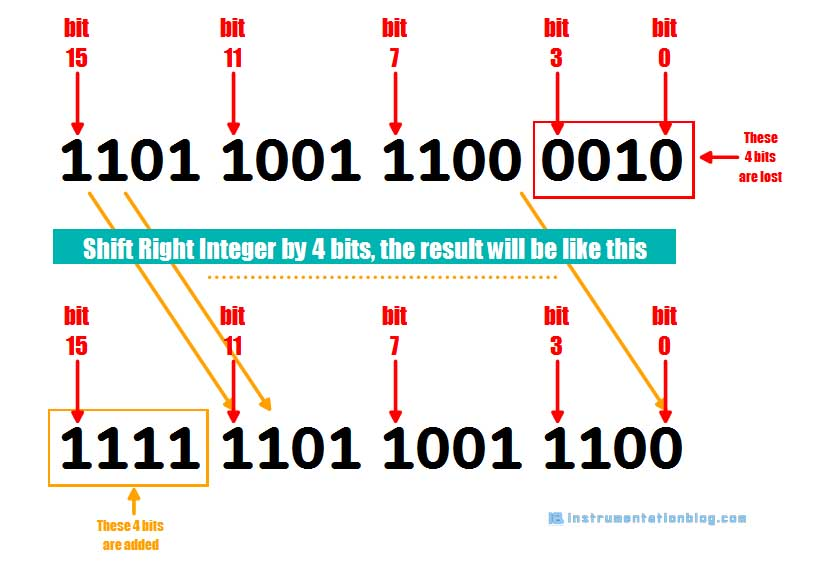#### Shift Right Double Integer (SHR_DI)

Shift Right Double Integer instruction is used to shift input IN bits 0 to 31 by N number of bits to the right. If the value for N is larger than 32 then it will be considered 32.

After shifting operation the vacant bits are filled with 0 if the input integer is positive and by 1 if the input integer is negative.

As an example, if the input number is 0111 1111 0101 0100 1100 0011 1001 1110 and N is 6. That means your output will be 0000 0001 1111 1101 0101 0011 0000 1110.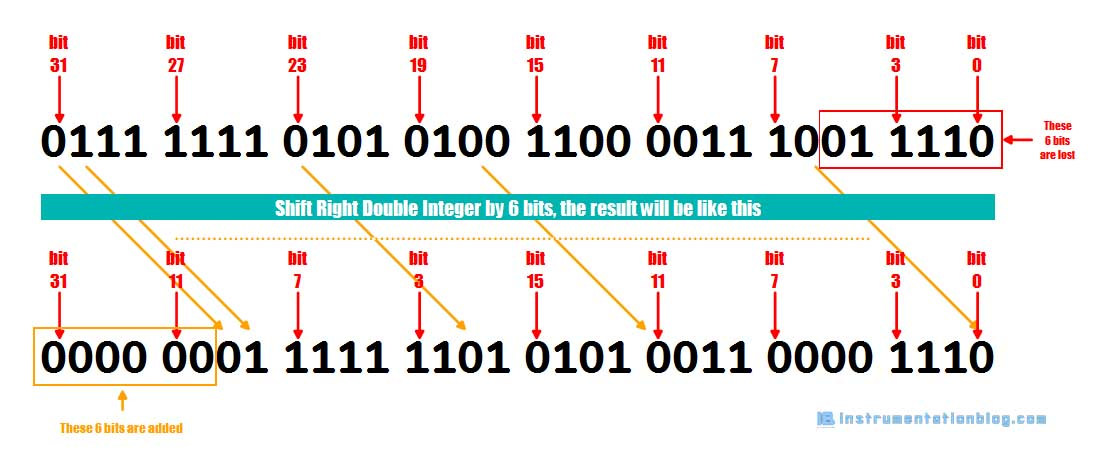#### Shift Left Word (SHL_W)

Shift Left Word instruction is used to shift input number IN bits 0 to 15 by N number of bits to the left. If the value for N is larger than 16 then the result will be 0.

After shifting operation vacant position will be filled with 0.

As an example, if the input number is 0101 1001 1111 0010 and N is 6. That means your output will be 0111 1100 1000 0000.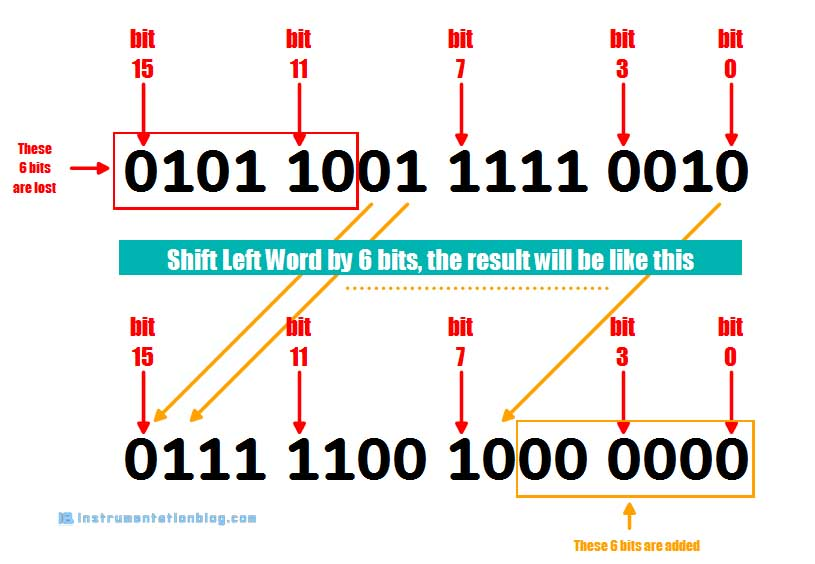If the input number is 1101 1001 1100 0010 and N is 16. That means your output will be 0000 0000 0000 0000.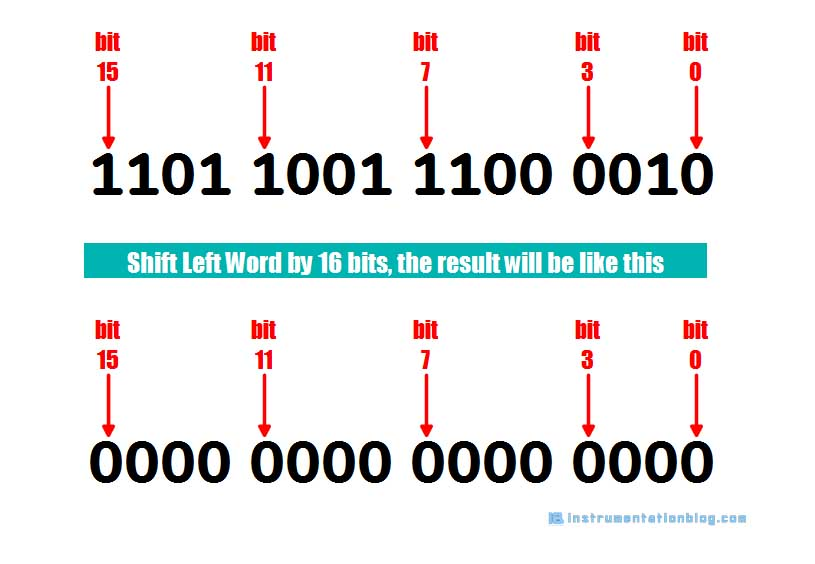#### Shift Left Double Word (SHL_DW)

Shift Left Double Word instruction is used to shift input number IN bits 0 to 31 by N number of bits to the left. If the value for N is larger than 32 then the result will be 0.

After shifting operation vacant position will be filled with 0.

#### Shift Right Word (SHR_W)

Shift Right Word instruction is used to shift input number IN bits 0 to 15 by N number of bits to the right. If the value for N is larger than 16 then the result will be 0.

After shifting operation vacant position will be filled with 0.

As an example, if the input number is 0101 1001 1111 0010 and N is 4. That means your output will be 0000 0101 1001 1111.If the input number is 1101 1001 1100 0010 and N is 16. That means your output will be 0000 0000 0000 0000.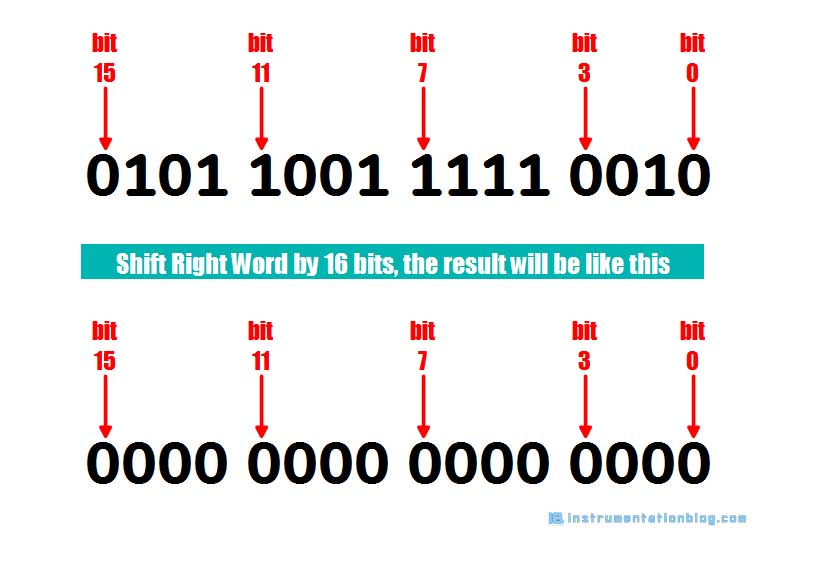#### Shift Right Double Word (SHR_DW)

Shift Right Word instruction is used to shift input number IN bits 0 to 31 by N number of bits to the right. If the value for N is larger than 32 then the result will be 0.

After shifting operation vacant position will be filled with 0.

### List of all Rotate instructions

• Rotate Left Double Word (ROL_DW)
• Rotate Right Double Word (ROL_RW)#### Rotate Left Double Word (ROL_DW)

Rotate Left Double Word instruction is used to rotate input number by N number of bits to the left direction. The bit positions shifted in from the right are assigned the logic states of the bits which were rotated out to the left.

If the value of N is larger than 32, then the input number is rotated by the ( (N-1)%32 + 1) bits.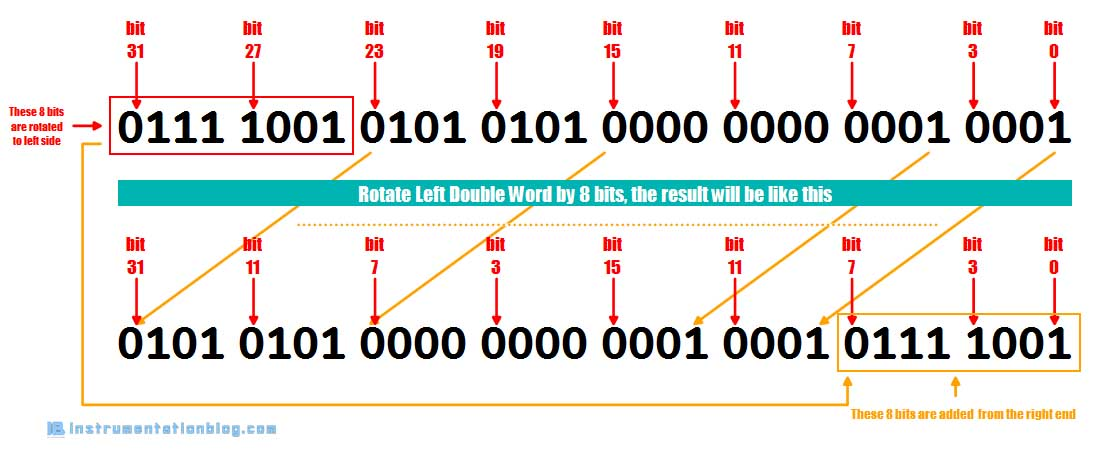#### Rotate Right Double Word (ROR_DW)

Rotate Right Double Word instruction is used to rotate input number by N number of bits in the right direction. The bit positions shifted in from the left are assigned the logic states of the bits which were rotated out to the right.

If the value of N is larger than 32, then the input number is rotated by the ( (N-1)%32 + 1) bits.⇒ Most popular 5 different types of plc programming languages.

⇒ Siemens PLC programming blocks.

⇒ 30+ most useful PLC communication protocols.

⇒ Basics of Different Types of PLC Modules

You can read more articles about Electrical and you can also find books that boost your knowledge in the field of Instrumentation